# MongoDB Aggregation Framework Cheat Sheet

## Importing sample data

This post is a quick introduction to the MongoDB Aggregation Framework. It’s a part of a mini-series called Mongo DB Cheatsheets

To follow the tutorial, you need to import the following data:

```[
{
"first_name": "Larry",
"last_name": "Freeman",
"networth": 340,
"investments": [
{
"name": "Oil Industry",
"year": 1990,
"value": 10
},
{
"name": "Bank Industry",
"year": 1995,
"value": 5
},
{
"name": "Bank Industry",
"year": 1997,
"value": 35
},
{
"name": "Publishing Industry",
"year": 1995,
"value": 130
},
{
"name": "Beer Industry",
"year": 2005,
"value": 40
},
{
"name": "Energy Industry",
"year": 2005,
"value": 55
}
]
},
{
"first_name": "Bill",
"last_name": "Freeman",
"networth": 80,
"investments": [
{
"name": "Oil Industry",
"year": 1992,
"value": 30
},
{
"name": "Oil Industry",
"year": 1995,
"value": 10
},
{
"name": "Bank Industry",
"year": 1995,
"value": 15
},
{
"name": "Bank Industry",
"year": 2005,
"value": 25
}
]
},
{
"first_name": "Jerry",
"last_name": "Whackman",
"networth": 190,
"investments": [
{
"name": "Publishing Industry",
"year": 1990,
"value": 130
},
{
"name": "Beer Industry",
"year": 1990,
"value": 40
},
{
"name": "Energy Industry",
"year": 2005,
"value": 15
}
]
},
{
"first_name": "Mary",
"last_name": "Whackman",
"networth": 280,
"investments": [
{
"name": "Oil Industry",
"year": 1997,
"value": 30
},
{
"name": "Bank Industry",
"year": 2005,
"value": 25
},
{
"name": "Auto Industry",
"year": 2008,
"value": 125
}
]
},
{
"first_name": "Kate",
"last_name": "Whackman",
"networth": 120,
"investments": [
{
"name": "Logistics Industry",
"year": 2017,
"value": 30
},
{
"name": "Bank Industry",
"year": 2005,
"value": 50
},
{
"name": "Auto Industry",
"year": 2008,
"value": 10
}
]
}
]
```

This sample is a list of 5 rich people from two families. The data contains their net worth and their investments over the years.

To import the data, open the MongoDB shell, select the database (starter) and do the import like this:

Now you are ready for some aggregations!

## MongoDB Aggregation Overview

You can think of aggregation as a pipeline where your data goes through a number of steps. The output of one step is the input of the next step.

## MongoDB Aggregation Quick example

From the list of rich people above, say, you want to get the people from the Whackman family, order the results by their net worth, and only display their name and net worth, ignoring other fields.

Then you can issue this aggregation query to achieve the result:

``` db.rich.aggregate([
{ \$match: { last_name: "Whackman" } },
{ \$sort: { networth: 1 } },
{ \$project: { _id: 0, first_name: 1, last_name: 1, networth: 1 } }
])```

As you can see, this aggregation has three stages:

• \$match: filter only matched documents. In the example, I filtered rich people who have a source as `self-made`
• \$sort: sort by field. (1 for ascending, -1 for descending)
• \$project: select fields to display (1 for showing, 0 for hiding)

The positions of the stages in the aggregation array determine the running order. That means in the example above, `\$match` runs first, then `\$set` and finally `\$project`.

## Using \$match for Filtering

You can use \$match as you can with `find`. This stage allows you to filter data to a smaller set.

In the list above, you can use \$match to filter people from one family only. For example, let’s get only people from the Freeman family:

``` db.rich.aggregate([
{ \$match: { last_name: "Freeman" } }
])```

You can also use \$match to do more complex filters like so:

Filter documents that made investments in years later than 2006. Notice that this filter still outputs the full documents with investments before 2006 (if any). The only requirement is that document has at least one investment after 2006.

``` db.rich.aggregate([
{ \$match: { "investments.year" : { \$gt: 2006} } }
])```

## Using \$project

You use \$project to modify fields, create new fields, and show and hide fields.

Here are some examples:

• Display people with their first name, last name and net worth only:
```db.rich.aggregate([
{ \$project: { _id: 0, first_name: 1, last_name: 1, networth: 1 } }
])```

In this example, for fields I want to show, I set the value to 1. I need to hide _id field explicitly. Other fields are hidden if there are no settings for them.

• Adding a new calculated field to the document

Let’s say there is a 10% tax applied to these rich people’s net worth and you want to display that field in the document. \$project would handle that easily:

```db.rich.aggregate([
{ \$project: { _id: 0, first_name: 1, last_name: 1, networth: 1, calculated_tax: {\$divide: ["\$networth", 10]} } }
])```

## Using \$unwind

I cannot put a better definition than the official of \$unwind so here it is:

Deconstructs an array field from the input documents to output a document for each element.

https://www.mongodb.com/docs/manual/reference/operator/aggregation/unwind/

For example, you have this document:

```{
"name": "Jane",
}```

After running \$unwind on this object, you will have two objects

```[{
"name": "Jane",
},
{
"name": "Jane",
"hobbies": "Swimming"
}]```

Let’s say the requirement is to show only investments in the Publishing Industry made by all of the people.

From the requirements, I’m going to write an aggregation pipeline with two stages: \$unwind and \$match:

```db.rich.aggregate([
{ \$unwind: "\$investments" },
{ \$match: { "investments.name" : "Publishing Industry" } }
])```

In this example, in the first steps, I used \$unwind to deconstruct the `investments` array. As a result, for each investment, I have one document. If I only run the \$unwind stage, here is the output:

As you can see, for one person (Larry Freeman for example), instead of one document, now he has multiple documents, each containing one of his investments.

The \$match stage filters out all the investments from industries that aren’t “Publishing Industry”. Thus, I got the result as expected.

## Using \$group

\$group is one of the most powerful operators in the aggregation framework. As the name suggests, you use \$group when you want to group documents that have common traits into one document.

Here are some important things you want to know about \$group:

• You need to define a “group key”, which is a common trait. This “group key” can be a field, a group of fields, or an expression
• You define the group key using _id

Let’s try an example with \$group. Let’s say I want to get the unique family names in the collections. From the beginning of this post, I already know that there are only two families: Freeman and Whackman

```db.rich.aggregate([
{ \$group: { _id: "\$last_name" } }
])```

### Using accumulators in \$group

Using accumulators helps you get some nice results with minimal code.

For example, you want to calculate the total net worth of each family. This is what you need to execute:

```db.rich.aggregate([
{ \$group: {_id: "\$last_name" , total_networth: { \$sum: "\$networth"}}}
])```

You can also use another function, \$avg to calculate the average net worth of each family:

```db.rich.aggregate([
{ \$group: {_id: "\$last_name" , total_networth: { \$avg: "\$networth"}}}
])```

## Using \$sample to extract a portion of a large dataset

There are times you have to work with large datasets. That means the document count is in the millions range. In such cases, you may want to work with a portion of that collection, says 1,000.

The `\$sample` operator helps you do that.

For example, the dataset in this post has 5 documents and I only want to work with 2, this is what I do:

```db.rich.aggregate([
{ \$sample: { size: 2 } }
])```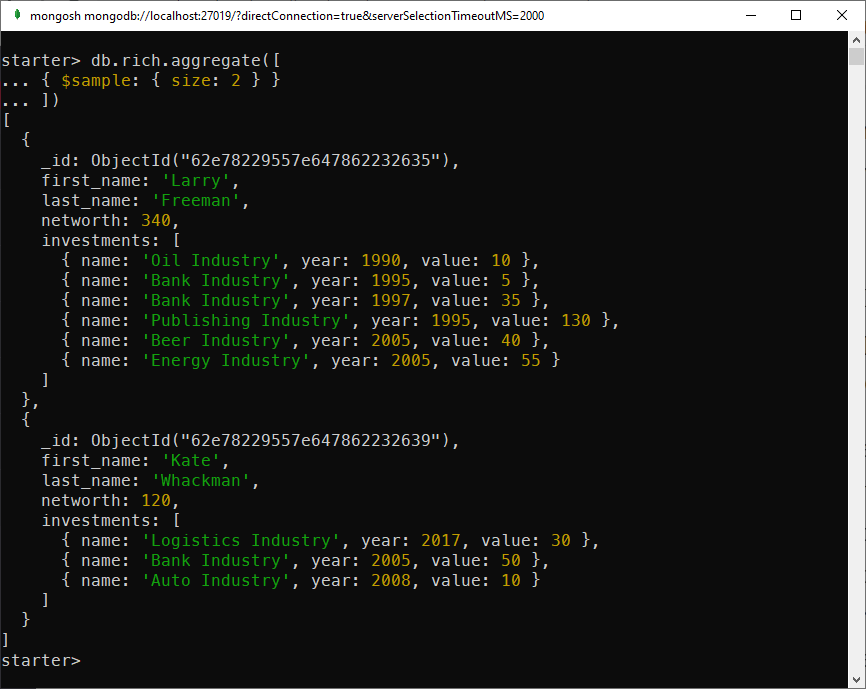Using \$sample to work with a subset of the dataset

## Practical MongoDB aggregation

In this section, we are going to solve some questions using aggregations.

### List the unique name of the industries

For this question, we are going to use \$unwind and \$group

```db.rich.aggregate([
{ \$unwind: "\$investments"},
{ \$group: {_id: "\$investments.name"}}
])```

### Which family invests most in the Oil industry?

For this question, we will do these steps:

• Use \$unwind to deconstruct the investments
• Use \$match to filter out other industries.
• Use \$group to group the family name and \$sum to calculate the total amount
```db.rich.aggregate([
{ \$unwind: "\$investments" },
{ \$match: { "investments.name" : "Oil Industry"} },
{ \$group: { _id: "\$last_name", total_investment: {\$sum: "\$investments.value" } } }
])```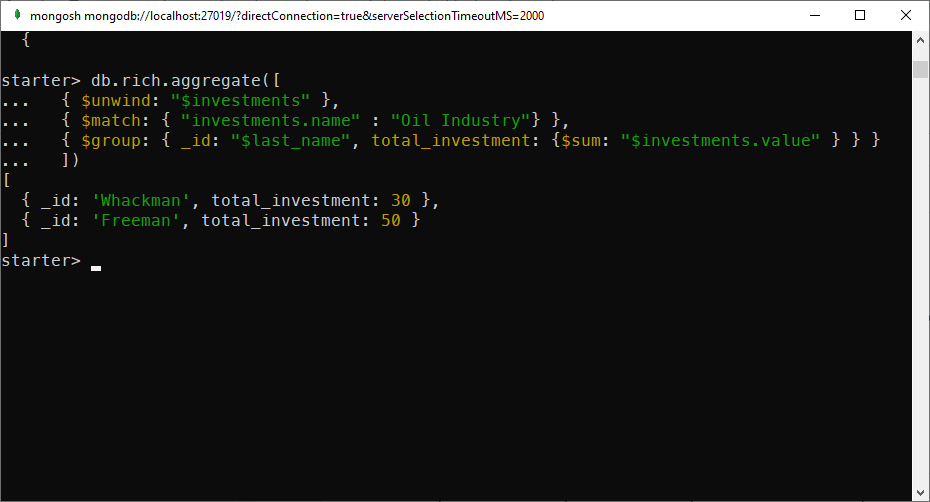Using aggregation to calculate total investments in Oil Industry by family

### Rank people by the total value of their investments

In this task, we need to calculate the sum of each person’s investments and then rank the value descendingly.

There are many ways to do this but this is one:

• First, \$unwind the investments array
• Then \$group by first name and last name, and use \$sum to calculate the total investments’ value
• Use \$sort to order the total value descendingly
```db.rich.aggregate([
{\$unwind: "\$investments"},
{ \$group: { _id: ["\$first_name", "\$last_name"], total_investment_value: { \$sum: "\$investments.value" } } },
{ \$sort : {"total_investment_value": -1} }
])```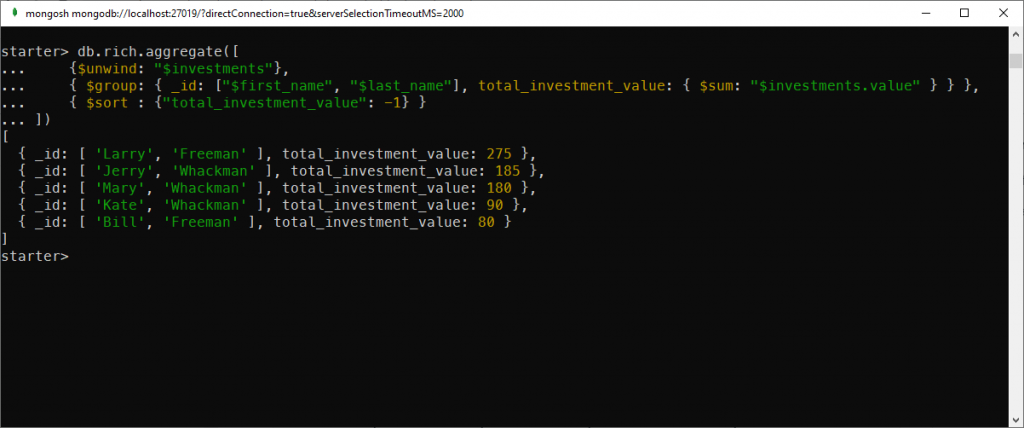Calculate the value of the total investments for all people and order the total descendingly

### Calculate the total net worth, and average net worth of each family and rank them by average net worth

At this point, you probably know what to do.

• Use \$group and \$sum, \$avg to calculate the requested values
• Use \$sort to rank the average net worth value
```db.rich.aggregate([
{ \$group: { _id: "\$last_name", total: {\$sum: "\$networth"}, average: {\$avg: "\$networth"}}},
{ \$sort: {"average": -1}}
])```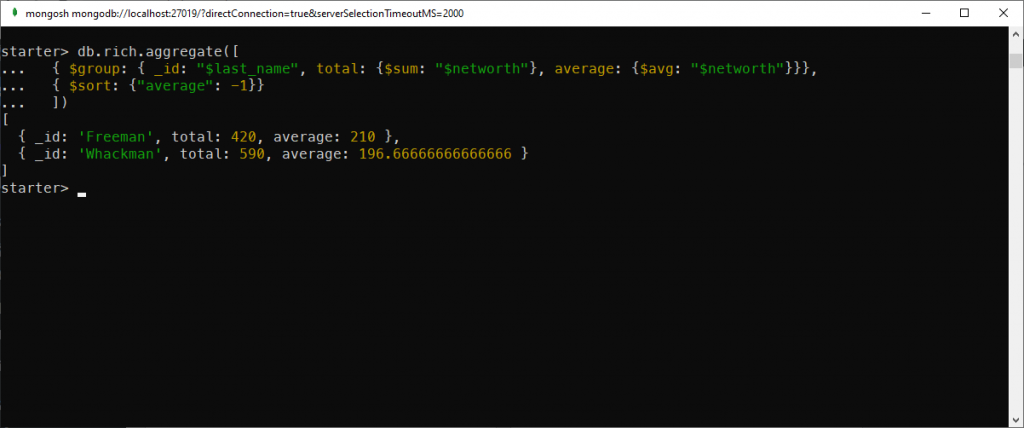Calculate the sum and average net worth by family

### Rank the top 3 investments by value.

This is yet another task we need to use \$unwind. Here are the steps:

• Use \$unwind to deconstruct the investment array
• Use \$sort to sort the investments by values
• Use \$limit to get only the top 3 items
```db.rich.aggregate([
{ \$unwind: "\$investments" },
{ \$sort: { "investments.value": -1 } },
{ \$limit: 3}
])```

### Which year has the most investment amount?

To solve this question, we need to use \$unwind, \$group, \$sort, and \$limit

```db.rich.aggregate([
{ \$unwind: "\$investments" },
{ \$group: { _id: "\$investments.year", amount: {\$sum: "\$investments.value"}} },
{ \$sort: {"amount": -1} },
{ \$limit: 1}
])```

### Count the number of people who have a total investment value greater than 50 in each family

To complete this request, we need to do quite many things:

• Use \$unwind to deconstruct the investment array
• Use \$group by _id and \$sum to calculate the total investment value of each person. In this step, we also use the accumulator `\$first` to extract the family name and store it in a field called `family_name`
• Use \$match to filter out people with less than 50 investment values
• Use \$group on last name and \$sum to count the number of the remaining people.
```db.rich.aggregate([
{ \$unwind: "\$investments" },
{ \$group: { _id: "\$_id", family_name: {\$first: "\$last_name"}, "invest_value": { \$sum: "\$investments.value"} } },
{ \$match: { "invest_value" : { \$gt: 50} } },
{ \$group: {_id: "\$family_name", "people_count": {\$sum: 1}}}
])```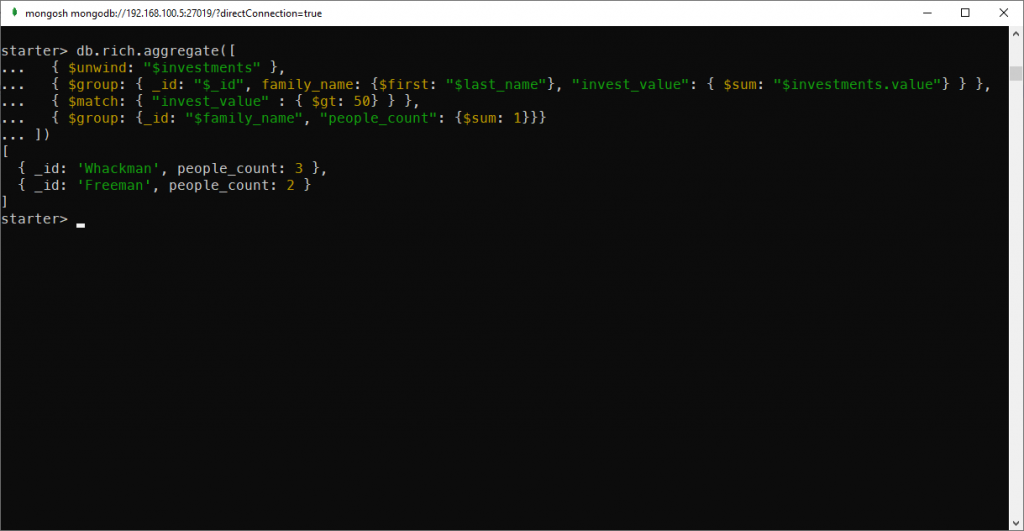Count the number of people per family that have an investment value of more than 50

## Conclusion

In this post, we have learned some of the most common usages of the MongoDB aggregation framework. This post is by no mean a complete reference. You need to refer to the official documentation for a complete list.